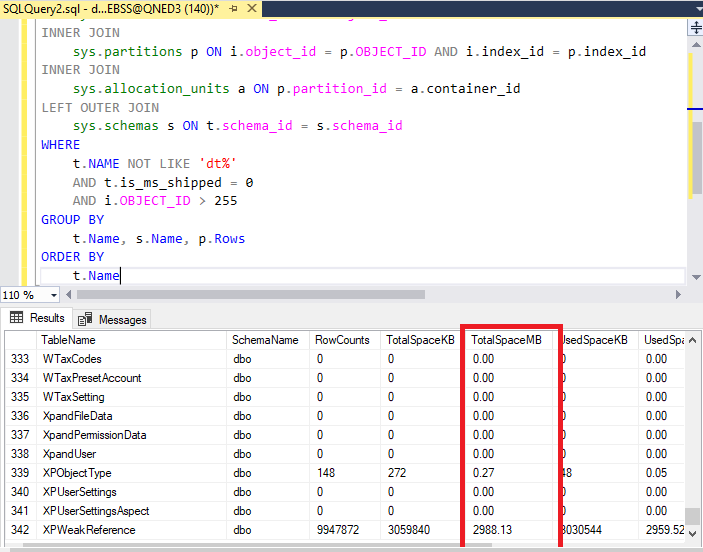If you find that database size is huge but no idea where the size is coming from, here is the script to find out.

Run below script in SQL Management Studio:

SELECT

t.NAME AS TableName,

s.Name AS SchemaName,

p.rows AS RowCounts,

SUM(a.total_pages) * 8 AS TotalSpaceKB,

CAST(ROUND(((SUM(a.total_pages) * 8) / 1024.00), 2) AS NUMERIC(36, 2)) AS TotalSpaceMB,

SUM(a.used_pages) * 8 AS UsedSpaceKB,

CAST(ROUND(((SUM(a.used_pages) * 8) / 1024.00), 2) AS NUMERIC(36, 2)) AS UsedSpaceMB,

(SUM(a.total_pages) - SUM(a.used_pages)) * 8 AS UnusedSpaceKB,

CAST(ROUND(((SUM(a.total_pages) - SUM(a.used_pages)) * 8) / 1024.00, 2) AS NUMERIC(36, 2)) AS UnusedSpaceMB

FROM

sys.tables t

INNER JOIN

sys.indexes i ON t.OBJECT_ID = i.object_id

INNER JOIN

sys.partitions p ON i.object_id = p.OBJECT_ID AND i.index_id = p.index_id

INNER JOIN

sys.allocation_units a ON p.partition_id = a.container_id

LEFT OUTER JOIN

sys.schemas s ON t.schema_id = s.schema_id

WHERE

t.NAME NOT LIKE 'dt%'

AND t.is_ms_shipped = 0

AND i.OBJECT_ID > 255

GROUP BY

t.Name, s.Name, p.Rows

ORDER BY

t.Name

2. Here is the sample. You will find that the most database usage from is from which table.3. From the data, we able to determine what to advice user to do, if we find that most of the size is coming from Audit Trail, we will suggest user to clear all the previous year's audit trail to reduce database size to save space in PC's storage or avoid running slow in the system. If we find that the database size is coming from other table which is for example Salesinvoices. Then you may seek for help from QNE Support.

Thank you.